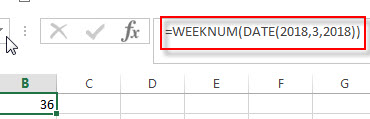# Excel WEEKNUM function

This post will guide you how to use Excel WEEKNUM function with syntax and examples in Microsoft excel.

### Description

The Excel WEEKNUM function returns the week number of a specific date, and the returned value is ranging from 1 to 53. And this function will start to count the week number from January 1 and the Sunday is the first day in one week by default.

The WEEKNUM function is a build-in function in Microsoft Excel and it is categorized as a DATE and TIME Function.

The WEEKNUM function is available in Excel 2016, Excel 2013, Excel 2010, Excel 2007, Excel 2003, Excel XP, Excel 2000, Excel 2011 for Mac.

### Syntax

The syntax of the WEEKNUM function is as below:

`=WEEKNUM (serial_number,[return_type])`

Where the WEEKNUM function arguments is:

• Serial_number –This is a required argument. An Excel date that you want to get the week number. The dates should be typed by the DATE function to make sure it is a valid Excel date. For example, use DATE(2018,3,20) to pass into the WEEKNUM function and then it will return the week number.
• return_type – This is an optional argument. A number that determines the first day of the week for the year, the default value is 1.
 Return_type Week begins on System 1 or omitted Sunday 1 2 Monday 1 11 Monday 1 12 Tuesday 1 13 Wednesday 1 14 Thursday 1 15 Friday 1 16 Saturday 1 17 Sunday 1 21 Monday 2

Note:

• If serial_number is out of range for the current date base value, a #NUM! Error is returned.
• If return_type is out of the range specified in the table above, a #NUM! Error is returned.
• A serial date is how the Microsoft Excel stores dates and it represents the number of days since 1900-01-01, so the January 1, 1900 date is serial number 1 by default.

### Excel WEEKNUM Function Examples

The below examples will show you how to use Excel WEEKNUM Function to return the week number from an Excel date.

1# get number of the week from a date value 2018/03/20, enter the following formula in Cell B1.

=WEEKNUM(DATE(2018,3,2018))### Related Functions

• Excel WEEKDAY function
The Excel WEEKDAY function returns a integer value representing the day fo the week for a given Excel date and the value is range from 1 to 7.The syntax of the WEEKDAY function is as below:=WEEKDAY (serial_number,[return_type])…
• Excel WORKDAY function
The Excel WORKDAY function returns a serial number that represents a date that is the indicated number of working days before or after the starting date you specified.The syntax of the WORKDAY function is as below:=WORKDAY(start_date, days, [holidays])…
• Excel DAY function
The Excel DAY function returns a day of a date (from 1 to 31).The DAY function is a build-in function in Microsoft Excel and it is categorized as a DATE and TIME Function.The syntax of the DAY function is as below:= DAY (date_value)…
• Excel EDATE function
TThe Excel EDATE function returns the serial number that represents the date that is a specified number of months before or after a specified date.The syntax of the EDATE function is as below:=EDATE (start_date, months)…
• Excel DATE function
The Excel DATE function returns the serial number for a date.The syntax of the DATE function is as below:= DATE (year, month, day)…

### More Excel WEEKNUM Function Examples

• Converting Week Number to Date
You need to create a formula based on the MAX function, the DATE function and the WEEKDAY function to convert week number to a date in Excel.…